# Generate HDL Code for Simscape Models

This example shows how to generate HDL code for a halfwave rectifier model that uses Simcape™ blocks. Use the Simscape HDL Workflow Advisor to generate an HDL implementation model. You can then generate HDL code for the implementation model. See Get Started with Simscape Hardware-in-the-Loop Workflow.

### The Halfwave Rectifier Model

To open the half-wave rectifier model, at the MATLAB command prompt, enter:

```open_system('sschdlexHalfWaveRectifierExample') ```

Save this model locally as `HalfWaveRectifier_HDL` to run the workflow.

```open_system('HalfWaveRectifier_HDL') set_param('HalfWaveRectifier_HDL', 'SimulationCommand', 'Update') ```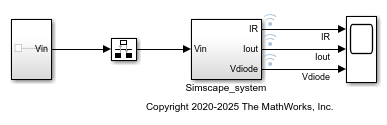At the top level of the model, a `Simscape_system` block models the half-wave rectifier algorithm. The model accepts a Sine Wave input, uses a Rate Transition block to discretize the continuous time input, and has a Scope block that calculates the output. To see the half-wave rectifier algorithm, double-click the `Simscape_system` subsystem.

```open_system('HalfWaveRectifier_HDL/Simscape_system') ```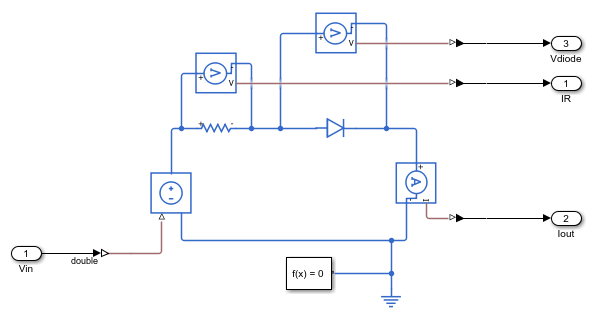The half-wave rectifier consists of a resistor, which is a linear block, and a diode, which is a switched linear block. The Simscape model is preconfigured for HDL compatibility. At the input and output port interfaces, the model has Simulink-PS Converter and PS-Simulink Converter blocks. The solver settings are configured for compatibility with Simscape HDL Workflow Advisor. If you open the Block Parameters dialog box for the Solver Configuration block, Use local solver is selected and `Backward Euler` is specified as the Solver type. See Get Started with Simscape Hardware-in-the-Loop Workflow.

To see the functionality, simulate the model and then open the Scope block.

```sim('HalfWaveRectifier_HDL') open_system('HalfWaveRectifier_HDL/Scope') ```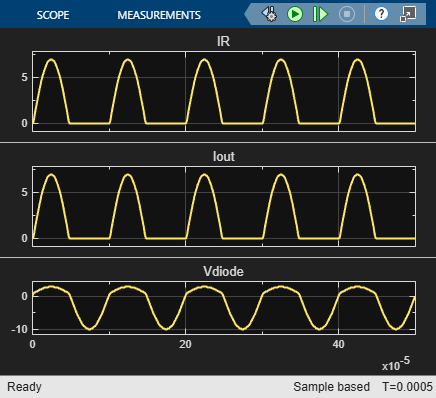### Run Simscape HDL Workflow Advisor

To generate an HDL implementation model from which you generate code, use the Simscape HDL Workflow Advisor. To open the Advisor, run this command:

```sschdladvisor('HalfWaveRectifier_HDL') ```
```### Running Simscape HDL Workflow Advisor for <a href="matlab:(HalfWaveRectifier_HDL)">HalfWaveRectifier_HDL</a> ```

To run the workflow and compare functionality of the HDL implementation model with the original Simscape algorithm, select the Generate implementation model step, and then select the Generate validation logic for the implementation model check box. Use a Validation logic tolerance of `0.001`. Right-click the Generate implementation model step and select Run to Selected Task.

The Advisor generates an HDL implementation model and a state-space validation model. The implementation model has the same name as the original Simscape model and uses the prefix `gmStateSpaceHDL_`. The state-space validation model has the same name as the implementation model and uses the postfix `_vnl`.

### Open and Examine HDL Implementation Model

In the Generate implementation model task, click the link to open the implementation model. The model contains a `Simscape_system` subsystem that contains a `HDL Subsystem` block. The `HDL Subsystem` models the state-space representation that you generated from the Simscape model.

```open_system('gmStateSpaceHDL_HalfWaveRectifier_HDL') open_system('gmStateSpaceHDL_HalfWaveRectifier_HDL/Simscape_system') set_param('gmStateSpaceHDL_HalfWaveRectifier_HDL','SimulationCommand','Update') ```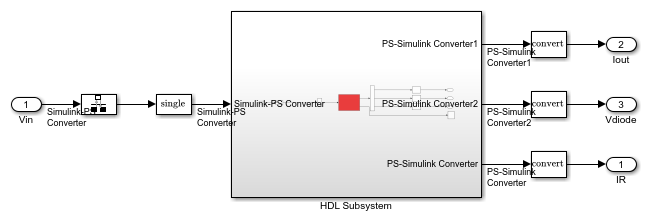The ports of this subsystem use the same name as the Simulink-PS Converter and PS-Simulink Converter blocks in your original Simscape model. If you navigate inside this subsystem, you see several delays, adders, and Matrix Multiply blocks that model the state-space equations.

```open_system('gmStateSpaceHDL_HalfWaveRectifier_HDL/Simscape_system/HDL Subsystem/HDL Algorithm') ```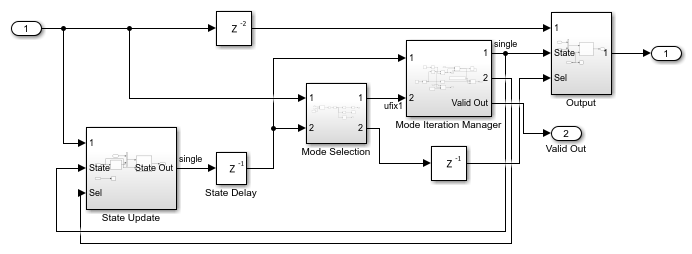To simulate the HDL Implementation model, enter tese commands. Open the Scope block to view results.

```sim('gmStateSpaceHDL_HalfWaveRectifier_HDL') open_system('gmStateSpaceHDL_HalfWaveRectifier_HDL/Scope') ```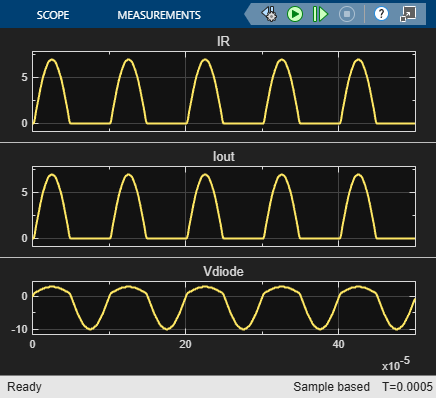HDL code is generated for the `HDL Subsystem` block inside this model.

### Validate HDL Algorithm

To compare functionality of the HDL implementation model with the original Simscape algorithm, open and simulate the state-space validation model.

```open_system('gmStateSpaceHDL_HalfWaveRectifier_HDL_vnl') sim('gmStateSpaceHDL_HalfWaveRectifier_HDL_vnl') ```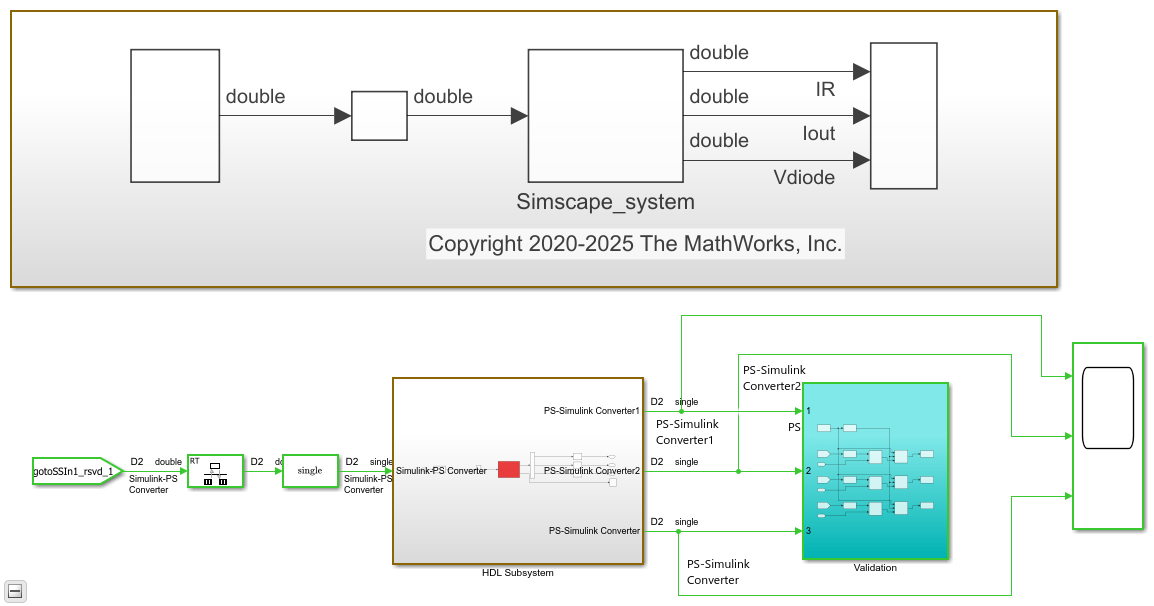The output of this model matches the original Simscape model. The simulation does not generate assertions, which indicates that the outputs match. For a more systemic verification, see Validate HDL Implementation Model to Simscape Algorithm.

In some cases, your Simscape algorithm might not be compatible for generating an implementation model by using the Simscape HDL Workflow Advisor. In such cases, running certain tasks in the Advisor can result in the task to fail. To learn how you can make it HDL compatible, see:

### Generate HDL Code and Validation Model

The HDL model and subsystem parameter settings are saved using this command:

```hdlsaveparams('gmStateSpaceHDL_HalfWaveRectifier_HDL'); ```
```%% Set Model 'gmStateSpaceHDL_HalfWaveRectifier_HDL' HDL parameters hdlset_param('gmStateSpaceHDL_HalfWaveRectifier_HDL', 'FloatingPointTargetConfiguration', hdlcoder.createFloatingPointTargetConfig('NativeFloatingPoint' ... , 'LatencyStrategy', 'MIN') ... ); hdlset_param('gmStateSpaceHDL_HalfWaveRectifier_HDL', 'HDLSubsystem', 'gmStateSpaceHDL_HalfWaveRectifier_HDL/Simscape_system/HDL Subsystem'); hdlset_param('gmStateSpaceHDL_HalfWaveRectifier_HDL', 'MaskParameterAsGeneric', 'on'); hdlset_param('gmStateSpaceHDL_HalfWaveRectifier_HDL', 'Oversampling', 60); % Set SubSystem HDL parameters hdlset_param('gmStateSpaceHDL_HalfWaveRectifier_HDL/Simscape_system/HDL Subsystem', 'FlattenHierarchy', 'on'); hdlset_param('gmStateSpaceHDL_HalfWaveRectifier_HDL/Simscape_system/HDL Subsystem/HDL Algorithm/Mode Selection/Generate Mode Vector', 'Architecture', 'MATLAB Datapath'); ```

The model uses single data types and generates HDL code in `Native Floating Point` mode. Floating-point operators can introduce delays. Because the design contains feedback loops, to allocate sufficient delays for the operators inside the feedback loops, the model uses clock-rate pipelining in conjunction with a large value for the Oversampling factor. An Oversampling factor of `60` and the clock-rate pipelining optimization is saved on this model.

Before you generate HDL code, enable generation of the validation model. The validation model compares the output of the generated model after code generation and the original model. To learn more, see Generated Model and Validation Model.

Run these commands to save validation model generation settings on your Simulink model:

```HDLmodelname = 'gmStateSpaceHDL_HalfWaveRectifier_HDL'; hdlset_param(HDLmodelname, 'TargetDirectory', 'C:/Temp/hdlsrc'); hdlset_param(HDLmodelname, 'GenerateValidationModel', 'on'); ```

To generate HDL code, run this command:

```makehdl('gmStateSpaceHDL_HalfWaveRectifier_HDL/HDL Subsystem'); ```

The generated HDL code and validation model are saved in `C:/Temp/hdlsrc` directory. The generated code is saved as `HDL_Subsystem_tc.vhd`. To open the validation model, click the link to `gm_gmStateSpaceHDL_HalfWaveRectifier_HDL_vnl.slx` in the code generation logs in the Command Window.

Open the `Compare` block at the output of `HDL Subsystem_vnl` subsystem of the validation model. Then, open the `Assert_Out1` block. To see the simulation results after HDL code generation, open the `Compare: Out1` Scope block. The top graph represents the output of the generated model, and the middle graph represents the output of the implementation model. The botton graph calculates the difference between outputs of both models. As the outputs match, the error is zero.